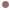Autres Articles de :
The challenging systems

# A Chua electronic circuit

Christophe LETELLIER
13/10/2009

The electronic circuit is composed by a network of linear passive elements connected to a nonlinear active component called Chua’s diode .

The set of differential equations that describes the circuit behavior iswhere,, L and R are the values of the passive linear elements, anddenotes the internal resistance of the inductor winding. The static nonlinearity of Chua’s diode is the piecewise linear function given byEstimated (by Unscented Kalman Filtering) physical values of the circuit are :R = 1673.6 ohms= 30.136 micro Farads= 185.66 micro FaradsL = 52.282 Henries. This is achieved not with a physical inductor but with a Gyrator= -1.365 mili smho (1/ohms)= -1.801 mili smho (1/ohms)= 1.739 volts= 0 ohms (because of gyrator).Experimental data from the Chua circuit.

Experimental data collected by Leonardo Torres can be dowloaded. There are four columns that are the time, X, Y, and Z, respectively. They were used to provide this representation of the double-scroll attractor (Fig. 1).Fig. 1 : Double scroll attractor reconstructed from experimental data collected by Leonorda Torres.

Observability coefficients are,,that leads to rank the variables according to their quality asL. A. B. Tôrres & L. A. Aguirre, PCCHUA --- A laboratory setup for real-time control and synchronization of chaotic oscillations, International Journal of Bifurcation and Chaos, 8 (15), 2349—2360, 2005.

 L. Torres & L. A. Aguirre, Inductorless Chua circuit, Electronics Letters, 36 (23), 1915—1916, 2000 for details.

### Documents

Experimental data from the Chua circuit.Zip · 210.9 ko
4517 - 20/02/20